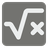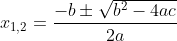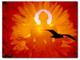Undefined
DE English FR PL UK ZH-HANS

A quadratic equation is represented as `ax2 + bx + c = 0`. The general formula to find its roots (x-values for which y = 0) is:This formula provides two roots: `x1, x2`. The value inside the square root, `b2-4ac` is known as the discriminant (`D`). Based on the value of the discriminant, a quadratic equation can have:

• two distinct real roots (`D > 0`);
• one real root (`D = 0`);
• no real roots (`D < 0`).

Your code must return Iterable containing two values: the roots `x1, x2`, sorted descending. If there's only one real root, both values will be the same. If there are no real roots, the Iterable should contain the string `"No real roots"`.

Input: Three integers (int).

Output: Tuple or other Iterable of two integers (int) or string (str).

Examples:

```assert list(quadratic_roots(1, -3, 2)) == [2, 1]
assert list(quadratic_roots(1, 0, -1)) == [1, -1]
assert list(quadratic_roots(1, 2, 1)) == [-1, -1]
assert list(quadratic_roots(1, 0, 1)) == ["No real roots"]
```

How it’s used: this function can be useful in mathematical computations, physics simulations, optimization problems, or anywhere quadratic equations are utilized.

Preconditions:

• a != 0;
• -109 <= a, b, c <= 109.37
Settings
Code:
Other:
Invalid hot key. Each hot key should be unique and valid
Hot keys:
•  to Run Code: to Check Solution: to Stop:
CheckiO Extensions

CheckiO Extensions allow you to use local files to solve missions. More info in a blog post.

In order to install CheckiO client you'll need installed Python (version at least 3.8)

Install CheckiO Client first:

`pip3 install checkio_client`

`checkio --domain=py config --key=`

Sync solutions into your local folder

`checkio sync`

(in beta testing) Launch local server so your browser can use it and sync solution between local file end extension on the fly. (doesn't work for safari)

`checkio serv -d`

Alternatevly, you can install Chrome extension or FF addon

`checkio install-plugin`
`checkio install-plugin --ff`
`checkio install-plugin --chromium`

Read more here about other functionality that the checkio client provides. Feel free to submit an issue in case of any difficulties.